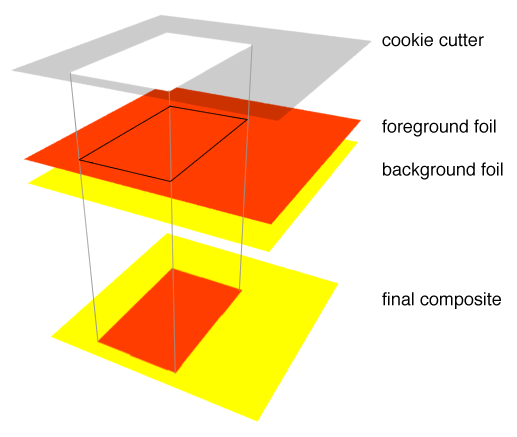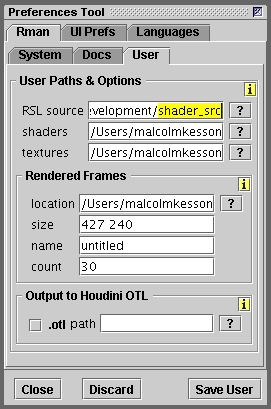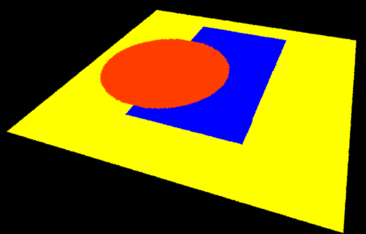#### Introduction

It is useful to think about a shading effect, say making a colored pattern, as a process of sequentially applying layers of colored foil to a surface. As each layer of foil is applied it is cut out by a digital "cookie-cutter".Figure 1

Figure 1 shows a "cookie-cutter" and foreground and background colored "foils". The cookie-cutter can also be thought of as a mask that allows one layer of color to be composited onto another layer of color. The cookie-cutter ie. mask, defines areas of transparency and opacity. Opaque areas allow parts of a foreground foil to overlay a background foil.

Listing 1 provides the code for a function that returns 0 or 1 depending on whether an location (`x`, `y`) is inside or outside a rectangle defined by its `top`, `left`, `bottom` and `right` edges. The function should be saved in a file called "lib.h".

Listing 1

 ```float rectangle(float top, left, bottom, right, x, y) { float result = 0; if(x >= left && x <= right && y >= top && y <= bottom) result = 1; return result; }```

The value retured from the function is used by `mix`() in listing 2 to overlay or composite the final color.

The source for a simple layered shader is shown below in listing 2.

Listing 2

 ```#include "lib.h" surface layering1(float Kfb = 1, top = 0.4, left = 0.4, bottom = 0.6, right = 0.6; color foreground = color(1,0.239,0)) { color surfcolor; float mask; /* STEP 1 Set the apparent surface opacity. */ Oi = Os; /* STEP 2 Composite a foreground color over a */ /* background color - in this instance */ /* Cs is the background color. */ mask = rectangle(top, left, bottom, right, s, t); surfcolor = mix(Cs, foreground, mask); /* STEP 3 No need to use Cs again because it was */ /* used it in step 2. */ Ci = Oi * surfcolor * Kfb; }```

If you are using Cutter to compile the RSL source code make sure that both the "lib.h" and "layering1.sl" are in the shader source code directory specified in Cutter's Preferences - figure 2. If you are not using Cutter the full path to the "lib.h" file must be specfied by the #include statement.Figure 2

#### Multiple Layers

By repeating step 2 several times, for example,

```    mask = rectangle(0.1, 0.3, 0.8, 0.7, s, t);

mask = circle(0.3, 0.5, 0.25, s, t);

a shader can give the illusion the surface to which it is assigned has multiple colored layers - figure 3.Figure 3

A function called `circle`() was implemented in the "lib.h" file as follows,

Listing 3

 ```float circle(float locx, locy, radius, x, y) { float result = 0; float d = distance(point(locx,locy,0), point(x,y,0)); if(d <= radius) result = 1; return result; }```